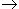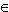Items‎ > ‎

### @ Parallel Bubble Sort and Theorem Z

 :If a network with n input elements sorts all 2^n sequences of 0’s and 1’s into non-decreasing order, it will sort any arbitrary sequence of n integers into non-decreasing order.Proof of Knuth’s 0-1 principal:If f(x) is any monotonic function, with f(x) <= f(y) whenever x <= y.When f(x) is monotonic min(f(x),f(y)) = f(min(x,y)) and max(f(x),f(y)) = f(max(x,y))     and if a given network N transforms (x_1,...,x_n) into (y_1,...,y_n): N(x_1,...,x_n) = (y_1,...,y_n)then that the network will transform f(x_1),...,f(x_n) into f(y_1),...,f(y_n): N( f(x_1),...,f(x_n) ) = ( f(y_1),...,f(y_n) )Proof:Suppose the network has only one comparator C comparing elements at indexes i and j the i’th element will be min( f(x_i), f(x_j) ) = f( min(x_i, x_j) ).the j’th element will be max( f(x_i), f(x_j) ) =  f( max(x_i,x_j) ).all other elements k will be f(x_k).  The result will be the same as applying the network to (x_1, … , x_n), and the applying function f to the result. This proves the base case of an induction. Assume that the above holds for a network of q comparators. Then add a comparator between any outputs of the network of q comparators to get a q+1 comparator network. The effect of this addition is exactly the same as in the base case where there is only one comparator, this shows that the order of the application of the network, and of the monotonic function are interchangeable for a q+1 comparator network.By induction this shows that the application of a comparator network to some input (x_1,...,x_n) and the application of a monotonic function to that network can commute. N( f(x) )  =  f( N(x) ) Suppose there is some sequence a=(a_1,... , a_n ) that network N fails to sort. Then N(a)=(b_1,... , b_n ), and there is at least one position i such that b_i > b_i+1. Choose the first such position. Define monotonic function f that maps all numbers less that b_i to 0 and all numbers greater than or equal to b_i to 1. Since N(f(a))=f(N(a)), applying the network to the output of the function will produce an unsorted sequence. This means if there exists an integer sequence not sorted by N, then f(a) will be a bit sequence not sorted by N. Equivalently if there is no bit sequence that is left unsorted by N, then there can be no integer sequence that is left unsorted by N. References:Knuth Searching & Sorting Pg223.http://www.iti.fh-flensburg.de/lang/algorithmen/sortieren/networks/nulleinsen.htm//Compare-Exchangedefine CE(A,B): if ( A > B ): temp = A A = B B = ADefinition:  A comparator is a mapping [i : j] : A^n[i:j](a)i =  min(a_i, a_j)[i:j](a)j =  max(a_i, a_j)[i:j](a)k =  a_k  for all k  with  k != i,  k != jfor all a in A^n.Problem statement: Given a sequence S of n integers produce a sequence S’ such that each integer present in S is present in S’, and occurs as many times in S’ as in S. Additionally, every element at some index i of S’ must be less than or equal to the element at index i+1 of S’ if such an index exists.  Algorithm Definition:Input: A sequence S of n integers, A sequence P of n unique processors. A processor in P at index i may communicate with processors at indexes i-1 (if i-1>0) and i+1 (if i+1